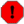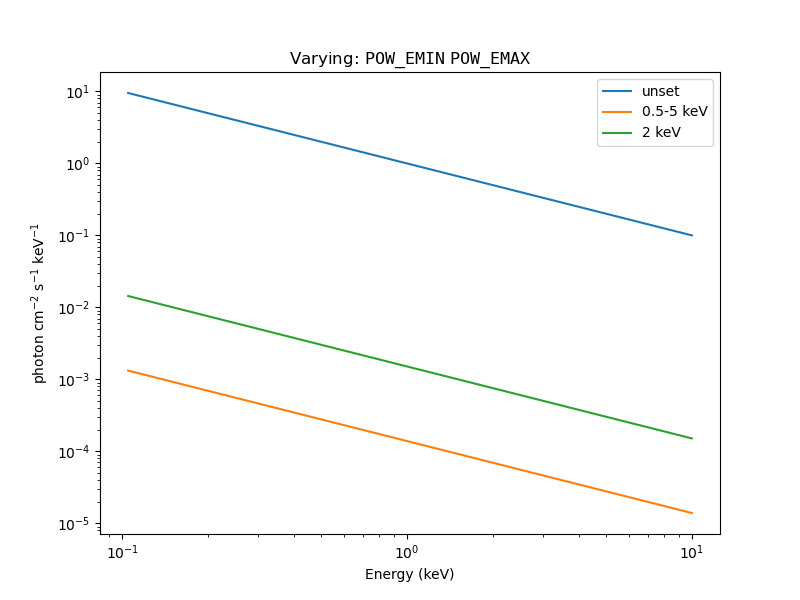# How do I change an XSPEC xset variable?

A number of XSPEC models use "environment variables" to modify their behavior via the xset command. The set_xsxset and get_xsxset routines let you set and inspect these values from Sherpa.

The normalisation of the xspowerlaw model defaults to defining the amplitude at 1 keV, but this can be changed by setting the POW_EMIN and POW_EMAX variables, as described at the XSPEC model description. Here we show how to make these changes from within Sherpa:

```% sherpa
-----------------------------------------------------
Welcome to Sherpa: CXC's Modeling and Fitting Package
-----------------------------------------------------
Sherpa 4.14.0

sherpa> pl = xspowerlaw.pl
sherpa> pl.norm
<Parameter 'norm' of model 'pl'>
sherpa> pl.norm.val
1.0
sherpa> get_xsxset("POW_EMIN")
''
sherpa> get_xsxset("POW_EMAX")
''
```Be careful with model caching

In order to reduce the time taken to fit spectra, Sherpa tries to "cache" model evaluations (that is, it looks to see if the model has already been evaluated with the set of parameter values, and if it has it can return the previously-calculated results). Unfortunately there is no way for the model to know whether "external" settings relevant to it - such as POW_EMIN and POW_EMAX in this case - have been changed, which can result in the new settings not being picked up.

We therefore turn off the cache for this model (otherwise all three models would evaluate to the same values below).

```sherpa> pl._use_caching
True
sherpa> pl._use_caching = False
```

After changing the cache setting, we can evaluate the models on our grid:

```sherpa> ebins = np.arange(0.1, 10, 0.01)
sherpa> elo = ebins[:-1]
sherpa> ehi = ebins[1:]
sherpa> y1 = pl(elo, ehi)
sherpa> set_xsxset("POW_EMIN", "0.5")
sherpa> set_xsxset("POW_EMAX", "5")
sherpa> y2 = pl(elo, ehi)
sherpa> set_xsxset("POW_EMIN", "2")
sherpa> set_xsxset("POW_EMAX", "2")
sherpa> y3 = pl(elo, ehi)
sherpa> set_xsxset("POW_EMIN", "")
sherpa> set_xsxset("POW_EMAX", "")
```

In the above, we have evaluated the pl model over the energy range 0.1 to 10 keV (with a bin width of 0.01 keV), three times:

1. y1 uses the default settings, so it should have a flux of 1 photon/cm2/s at 1 keV;
2. y2 has POW_EMIN set to 0.5 and POW_EMAX set to 5, so the model norm value now refers to the integrated flux over the range 0.5 to 5 keV (in units of 10-12 ergs/cm2/s);
3. and y3 has POW_EMIN and POW_EMAX both set to 2, so the normalisation now refers to the value at 2 keV.

After evaluating the models we set the values back to their default settings. These curves can be plotted to show the change in amplitudes (note that we divide by the bin width before plotting, since the XSPEC models evaluate to photon cm-2 s-1):

```sherpa> fig = plt.figure(figsize=(8, 6))
sherpa> emid = (elo + ehi) / 2
sherpa> plt.plot(emid, y1 / 0.01, '-', label='unset')
sherpa> plt.plot(emid, y2 / 0.01, '-', label='0.5-5 keV')
sherpa> plt.plot(emid, y3 / 0.01, '-', label='2 keV')
sherpa> plt.xscale('log')
sherpa> plt.yscale('log')
sherpa> plt.legend()
sherpa> plt.ylabel('photon cm\$^{-2}\$ s\$^{-1}\$ keV\$^{-1}\$')
sherpa> plt.xlabel('Energy (keV)')
sherpa> plt.title(r'Varying: \${\tt POW\_EMIN}\$ \${\tt POW\_EMAX}\$')
```

which creates the following plot: